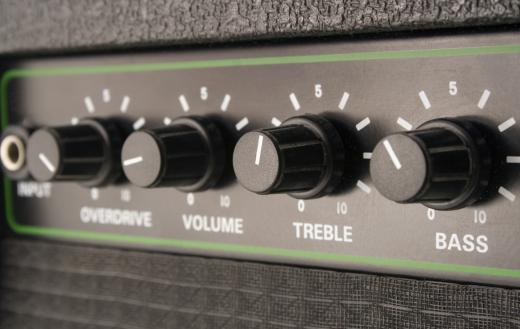# What is Analog Signal Processing?

Jason C. Chavis
Jason C. Chavis

Analog signal processing is the mathematical operation or analysis of analog signals through analog means. This can be conducted in either a discrete or continuous time frame and represents the main way in which these operations perform. Usually this processing involves some form of control, filtering, deblurring or denoising.

In order for the signal to be analog, it must have a continuous value. Basically, the signal must feature a time varying flow of information. Each fluctuation in the signal has important meaning, unlike digital, which deals with numeric representation. The most common context of analog is in electronics, when a signal is sent as physical information.An amplifier converts signals from guitar pickups into sound.

In terms of audio, analog signal processing is responsible for the changes in bass, treble and volume controls. Video and television use the processing techniques to control the picture's tint. In each of these cases, the voltage and current are controlled by a series of capacitors, resistors, inductors and transistors.

Analog signal processing is defined in the concept of convolution. Convolution defines the parameters in which an input signal combines with the function of a system to determine the accurate output signal. With two analog waveforms, the convolution is the factor in which one of the waveforms is reversed and shifted. To calculate convolution, the first waveform is reversed and shifted to become identical to the second waveform. This creates the analog signal.

The concept of Fourier transformation is also important to analog signal processing. It defines the situation in which the operation transforms a complex analog signal into a series of individual components. This can occur over a period of time or frequency. An example of Fourier transformation is breaking down a musical chord into a number of individual notes.

Frequently, different types of signals are used in analog signal processing. These include sinusoids, impulses and steps. Sinusoids are the basic feature of processing. They demonstrate the deviations in analog through frequency and time variables. Impulses are signals that represent an infinite magnitude and width. The step signals are immediate pulses of information. They demonstrate the sudden input response, similar to the effects of turning a switch.

A number of everyday systems use the concept of analog signal processing. Changing the channel on an analog television requires the signal be filtered and processed in a certain way. AM/FM radio processes the various analog signals transported throughout the airwaves and creates the output information. Electric guitars also use an analog concept to produce music. The guitar's inductor turns the strings' vibration into electric current.